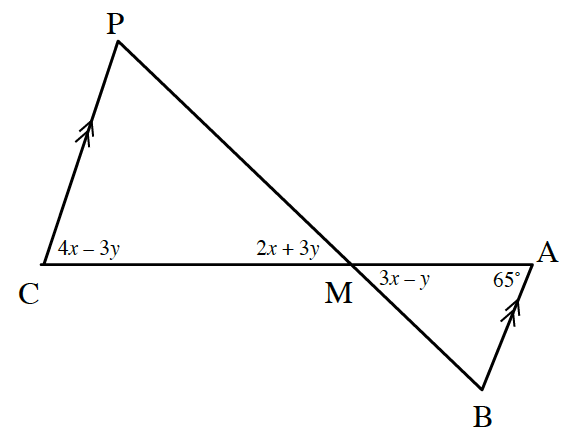### Home > CCA2 > Chapter Ch4 > Lesson 4.2.4 > Problem4-103

4-103.

Find the measure of $∠CPM$ in the diagram below. List any sub-problems that were necessary to solve this problem.Notice that $\overline{AB}\text{ and }\overline{CP}$ are parallel.

Remember geometric angle relationships.
If the lines are parallel, what must be true about the alternate interior angles?
What is always true about vertical angles?

$65=4x−3y$
$3x−y=2x+3y$

How can you find the measure of the third angle?

Write and solve a system of equations based on the properties.

Once you find $x$ and $y$, find the measures of the angles in $ΔCPM$.

$∠CPM=60°$